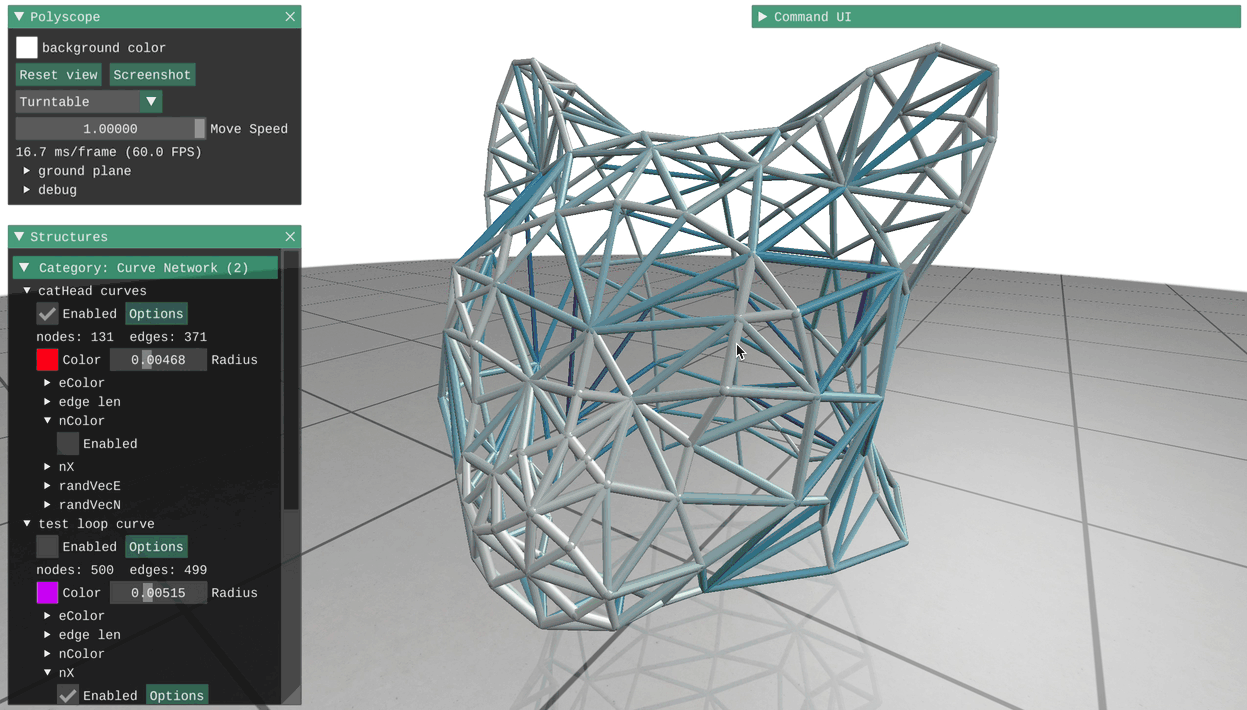# Curve Networks

Curve networks are collections of nodes sitting in space, connected by edges. In addition to displaying the nodes and edges of the network itself, Polyscope can show any number of scalar, vector, or color quantities associated with the nodes or edges of the network.

Try clicking on a node or edge to see the data associated with that point!### Registering a curve network

Curve network structures can be registered with Polyscope by passing the node position and edge indices. There are also two helpers for constructing lines and loops which only require the node locations and automatically build connectivity.

As usual in Polyscope, the data adaptors allow these functions to accept a wide variety of data types as input– any nodes which are essentially a list of vectors and any edges which are a list of index tuples will work. The std::vector<> types in the example below are just one possibility.

#include "polyscope/curve_network.h"

polyscope::init();

std::vector<glm::vec3> nodes = /* some nodes */;
std::vector<std::array<size_t, 2>> edges = /* edges between nodes */;

polyscope::registerCurveNetwork("my network", nodes, edges);

// visualize!
polyscope::show();


CurveNetwork* polyscope::registerCurveNetwork(std::string name, const P& nodes, const E& edges)

Add a new curve network to polyscope

• nodes is the array of 3D point locations. The type should be adaptable to an array of float-valued 3-vectors. The length will be the number of nodes.

• edges is the array of edges, each of which is a pair of 0-based node indices node. The type should be adaptable to an array of size_t-valued 2-vectors. The length will be the number of edges.

Note: the inner vector type of the nodes input must be 3D dimensional, or you risk compiler errors, segfaults, or worse. If you want to register a 2D curve network, registerCurveNetwork2D exists with the same signature. See 2D data.

CurveNetwork* polyscope::registerCurveNetworkLine(std::string name, const P& nodes)

Add a new curve network to polyscope from a polyline of points. The connectivity will be automatically created to connect the points in order.

• nodes is the array of 3D point locations. The type should be adaptable to an array of float-valued 3-vectors. The length will be the number of nodes.

Note: the inner vector type of the points input must be 3D dimensional, or you risk compiler errors, segfaults, or worse. If you want to register a 2D curve network, registerCurveNetworkLine2D exists with the same signature. See 2D data.

CurveNetwork* polyscope::registerCurveNetworkLoop(std::string name, const P& nodes)

Add a new curve network to polyscope from a closed loop of points. The connectivity will be automatically created to connect the points in order.

• nodes is the array of 3D point locations. The type should be adaptable to an array of float-valued 3-vectors. The length will be the number of nodes.

Note: the inner vector type of the points input must be 3D dimensional, or you risk compiler errors, segfaults, or worse. If you want to register a 2D curve network, registerCurveNetworkLoop2D exists with the same signature. See 2D data.

### Updating a curve network

The locations of the nodes in a curve network can be updated with the member function updateNodePositions(newPositions). All quantities will be preserved. Changing the connectivity or number of nodes/edges is not supported, you will need to register a new curve network (perhaps with the same name to overwrite).

void CurveNetwork::updateNodePositions(const V& newPositions)

Update the node positions in a curve network structure.

• newPositions is the vector array of 3D node locations. The type should be adaptable to an array of float-valued 3-vectors. The length must be equal to the current number of nodes.

Note: updatePointPositions2D exists with the same signature. See 2D data.

### Options

Parameter Meaning Getter Setter Persistent?
enabled is the structure enabled? bool isEnabled() setEnabled(bool newVal) yes
radius size of rendered points and lines double getRadius() setRadius(double newVal, bool isRelative=true) yes
color default color the curve network glm::vec3 getColor setColor(glm::vec3 newVal) yes
material what material to use std::string getMaterial() setMaterial(std::string name) yes

(all setters return this to support chaining. setEnabled() returns generic setter, so chain it last)